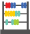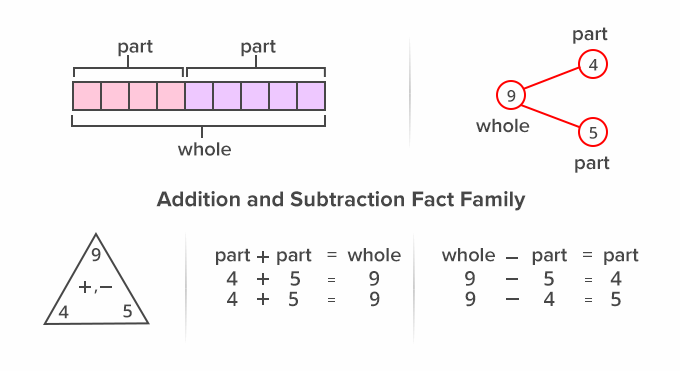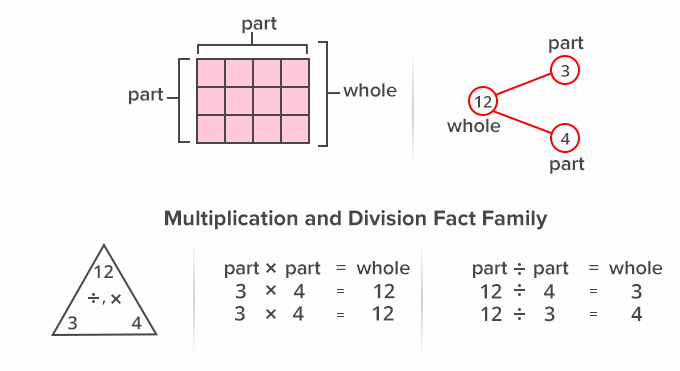# Fact Family - Definition with Examples

The Complete K-5 Math Learning Program Built for Your Child

• 30 Million Kids

Loved by kids and parent worldwide

• 50,000 Schools

Trusted by teachers across schools

• Comprehensive Curriculum

Aligned to Common Core

##Let's learn!

What is a fact family?
In math, a fact family can be defined as a group of math facts or equations created using the same set of numbers. The fact family shows the relationships between the three numbers involved.
In an addition and subtraction fact family, there are four addition and subtraction sentences created using three numbers.

Here, for instance, a fact family has been created using the numbers 9,4 and 5 where 4 and 5 are parts, while 9 is the whole.Similarly, in a multiplication and division fact family, there are multiplication and division sentences created using three numbers.

Here, for instance, a fact family has been created using the numbers 12, 4 and 3 where 4 and 3 are parts, while 12 is the whole.Fun Facts Fact families help us to understand the relationship between operations in math.

##Let's sing!

Three numbers – it’s a family bond!
Just multiply or divide to respond.
Or whether you add or subtract,
In the end, you get a family of facts!

##Let's do it!

Instead of handing out math worksheets on fact family, play a game with your children using jelly beans. Give jelly beans  in a set of three numbers, say 2, 3 and 5. Ask them to create an addition and subtraction fact family using the jelly beans. Repeat for multiplication and division fact family.

##Related math vocabulary

Won Numerous Awards & Honors